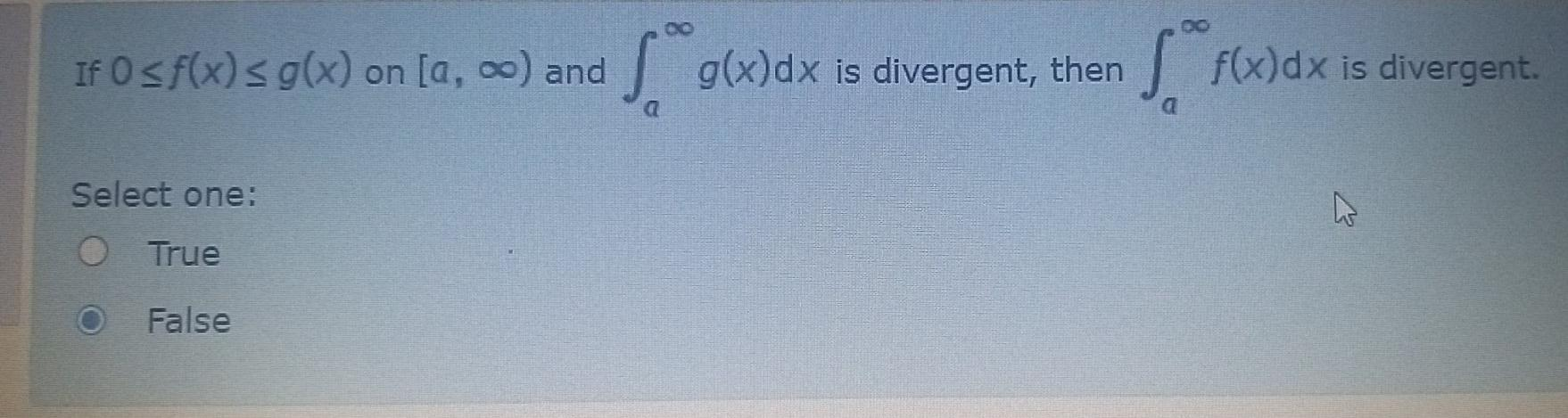### Create an Account

Home / Questions / If O sf(x) g(x) on [a, o) and Sºg()dx is divergent, then SF()dx is divergent Select one: True False

# If O sf(x) g(x) on [a, o) and Sºg()dx is divergent, then SF()dx is divergent Select one: True False

If O sf(x) g(x) on [a, o) and Sºg()dx is divergent, then SF()dx is divergent Select one: True FalseApr 26 2021 View more View LessSubscribe To Get Solution